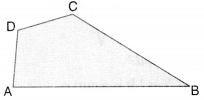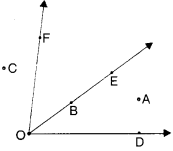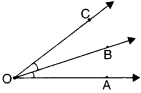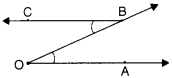NCERT Solutions for Class 6 Maths Chapter 4 Basic Geometrical Ideas Ex 4.3 are part of NCERT Solutions for Class 6 Maths. Here we have given NCERT Solutions for Class 6 Maths Chapter 4 Basic Geometrical Ideas Ex 4.3.

 Board CBSE Textbook NCERT Class Class 6 Subject Maths Chapter Chapter 4 Chapter Name Basic Geometrical Ideas Exercise Ex 4.3 Number of Questions Solved 3 Category NCERT Solutions

## NCERT Solutions for Class 6 Maths Chapter 4 Basic Geometrical Ideas Ex 4.3

Question 1.
Name the angles in the given figure.Solution :
∠A or ∠DAB; ∠B or ∠ABC; ∠C or ∠BCD; ∠Dor ∠CDA.

Question 2.
In the given diagram, name the point (s)
(a) in the interior of ∠DOE
(b) in the exterior of∠EOF
(c) on ∠EOF.Solution :
(a) A
(b) C, A, D
(c) E, B,0, F.

Question 3.
Draw rough diagrams of two angles such that they have
(a) One point in common
(b) Two points in common
(c) Three points in common
(d) Four points in common
(e) One ray in common.
Solution :
(a)∠AOB and ∠BOC have one point O in common.
(b) ∠AOB and ∠OBC have two points O and B in common.(c) Not possible
(d) Not possible
(e)∠AOB and ∠BOC have one ray $$\overrightarrow { OB }$$ in common

We hope the NCERT Solutions for Class 6 Maths Chapter 4 Basic Geometrical Ideas Ex 4.3 help you. If you have any query regarding NCERT Solutions for Class 6 Maths Chapter 4 Basic Geometrical Ideas Ex 4.3, drop a comment below and we will get back to you at the earliest.